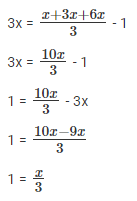# Dick is thrice as old as Tom and Harry - Quantitative Aptitude - CAT Age

## CAT 2020 - Slot 3 - Quantitative Aptitude - Age - Question 6 - Dick is thrice as old as Tom and Harry

Q.6: Dick is thrice as old as Tom and Harry is twice as old as Dick. If Dick’s age is 1 year less than the average age of all three, then Harry’s age, in years, is

Let’s consider Tom’s age to be ‘x’ years.

Dick is thrice as old as Tom,

Dick’s age = 3 × x = 3x

Harry is twice as old as Dick,

Harry’s age = 2 × 3x = 6x

Dick’s age is 1 year less than the average age of all three,

3x = Average(x, 3x, 6x) – 13 = x

x = 3

Harry’s age = 6x = 6 × 3 = 18 years.

### Past Year Question Paper & SolutionsCounselling Session
By IIM Mentor

#### Free Material Area